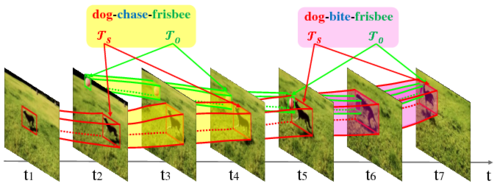### Main Task: Video Relation Detection

Video Visual Reltion Detection is a novel research problem that is beyond the recognition of single object entity. It requires the visual system to understand many perspectives of two entities, including appearance, action, intention, and interactions between them. Specifically, it aims to detect instances of visual relations of interest in a video, where a visual relation instance is represented by a relation triplet <subject,predicate,object> with the bounding-box trajectories of the subject and object during the relation happening (as shown in Figure 1). Note that the detection of social relations (e.g. “is parent of”) and emotional relations (e.g. “like”) is outside the scope of this task.Figure 1: Examples of visual relation instances in video. Adopted from " Video visual relation detection " (ACM MM'17)

The top-1 solution in VRU'19 challenge, including precomputed features and bounding box trajectories, are released at link1 (link2) to facilitate participation. Please kindly cite this paper if you use it in your work.

### Dataset

The VidOR dataset for this task consists of user-generated videos from Flickr and annotations on 80 categories of object (e.g. "adult", "child", "dog", "table"), 42 categories of verb (action) predicate (e.g. "watch", "grab", and "hug") and 8 categories of spatial predicate (e.g. "in front of", "inside", "towards"). In the annotations, each relation instance is labeled and localized in the same way as the instances shown in Figure 1 (e.g. "dog-chase-frisbee" from t2 to t4). The training/validation/testing splits are 7,000, 835 and 2,165 videos. The videos and annotations of the training and validation sets can be downloaded directly from here.

### Evaluation Metric

We adopt Average Precision (AP) to evaluate the detection performance per video and finally calculate the mean AP (mAP) over all testing videos as the ranking score. In particular, we calculate AP similarly to that in the Pascal VOC challenge. To match a predicted relation instance $(\langle s,p,o\rangle^p,(\mathcal{T}_s^p,\mathcal{T}_o^p))$ to a ground truth $(\langle s,p,o\rangle^g,(\mathcal{T}_s^g,\mathcal{T}_o^g))$, we require:

• their relation triplets to be exactly same, i.e. $\langle s,p,o\rangle^p = \langle s,p,o\rangle^g$;
• their bounding-box trajectories overlap s.t. $\text{vIoU}(\mathcal{T}_s^p, \mathcal{T}_s^g) \geq 0.5$ and $\text{vIoU}(\mathcal{T}_o^p, \mathcal{T}_o^g) \geq 0.5$;
• the minimum overlap of the subject trajectory pair and the object trajectory pair $\text{ov}_{pg}=\min{(\text{vIoU}(\mathcal{T}_s^p, \mathcal{T}_s^g), \text{vIoU}(\mathcal{T}_o^p, \mathcal{T}_o^g))}$ is the maximum among those paired with the other unmatched ground truths $\mathcal{G}$, i.e. $\text{ov}_{pg} \geq \text{ov}_{pg'} (g' \in \mathcal{G})$.
The term $\text{vIoU}$ refers to the voluminal Intersection over Union. While calculating the score, we only consider the top-200 predictions for each video.

The evaluation code used by the evaluation server can be found here.

### Submission Format

Due to the limitation of evaluation server, we have changed the submission format this year. The structure of submitted folder is shown as below:

result_xz
|——2400171624.xz/
|        |——2400171624
|——2400715506.xz/
|        |——2400715506
|...
|——14040960901.xz/
|        |——14040960901

Each file (such as 2400171624) is in the following JSON format and then compressed by XZ. The participators should submit a .zip folder (result_xz.zip). A sample submission file is available HERE.

The example above is illustrative. Comments must be removed in your submission.